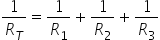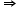Physics
Easy

Question

# A 47 Ω resistor, a 22 Ω resistor, and a 10 Ω resistor are connected in parallel. The total resistance is approximately

## 6 Ω1/6 Ω3 Ω1/3 ΩHint:

## The correct answer is: 6 Ω

### When a 47 Ω resistor, a 22 Ω resistor, and a 10 Ω resistor are connected in parallel, the total resistance is approximately 6Ω. The formula that can calculate the total resistance in a parallel circuit=Given, R1, R2, and R3 are 47Ω, 22Ω, and 10Ω respectively. So, we can calculate1/RT= 1/47+1/22+1/101/RT= 1/0.166= 6.02RT= 6.024 So the total resistance (RT) in the circuit is approx. 6 Ω.#### With Turito Foundation.#### Get an Expert Advice From Turito.